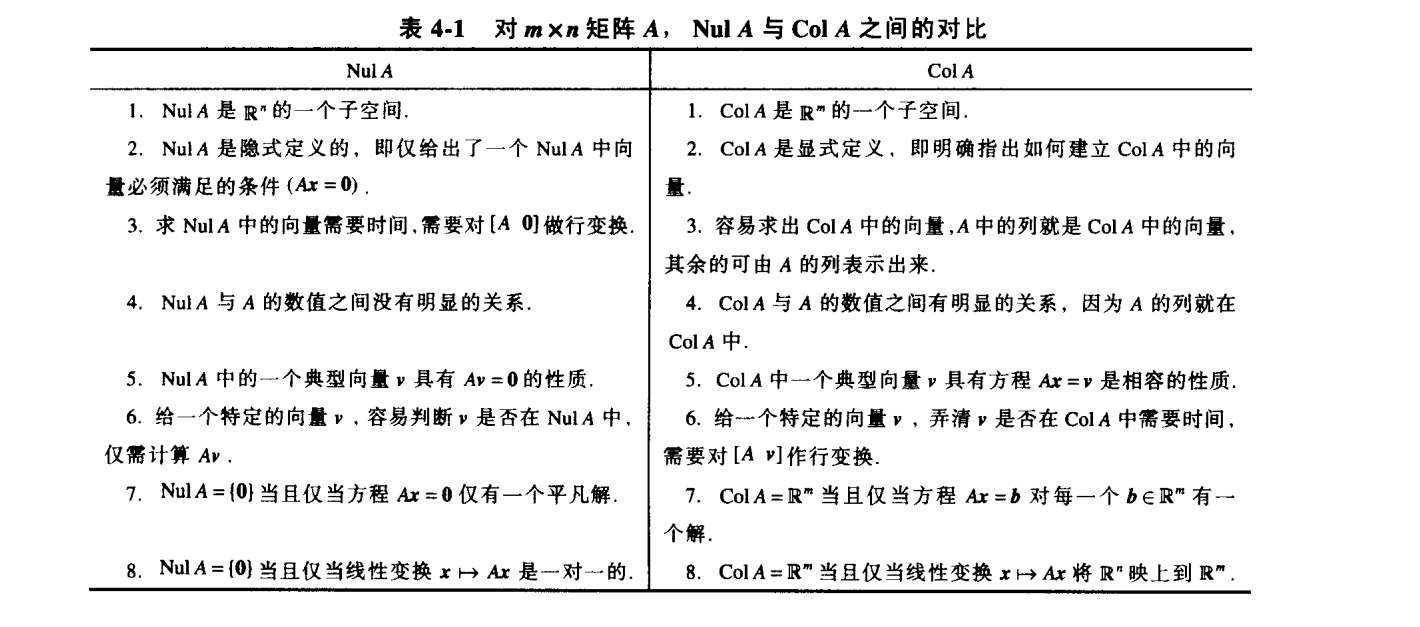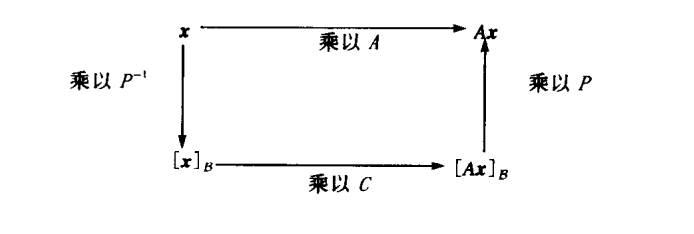# ch4 向量空间 ‘线性代数及其应用笔记’

具体数学

## 4.1 向量空间与子空间

1. u,v之和u+v仍在V中
2. u+v=v+u
3. (u+v)+w=u+(v+w)
4. V中存在一个零向量0，使u+0=u
5. 对V中每个向量u，存在V中向量-u，使得u+(-u)=0
6. u与标量C的标量乘法记为cu，仍在V中
7. c(u+v)=cu+cv
8. (c+d)u=cu+du
9. c(du)=(cd)u
10. 1u=u

1. V的零向量在H中
2. H对向量加法封闭
3. H对标量乘法封闭

## 4.2 零空间，列空间和线性变换

$m\times n$的矩阵A零空间写作NulA ，是齐次方程Ax=0的全体解的集合。
$NulA=\{ x:x\in\mathbb{R},Ax=0 \}$

$m \times n$矩阵的列空间是由A的列的所有线性组合组成的集合，若 $A=[a_1,...,a_n]$,则 $ColA=Span\{a_1,...,a_n\}$

Attention 如果一个矩阵不是方阵，它的零空间和列空间在完全不同的域， $m\times n$矩阵列空间在 $\mathbb{R}^m$，零空间在 $\mathbb{R}^n$1.1.png-163.9kB

1. T(u+v)=T(u)+T(v)
2. T(cu)=cT(u)

## 4.3 线性无关集和基

1. B是一线性无关集
2. $H=span\{b_1,...,b_p\}$

$S=\{v_1,...,v_p\}$是V中向量集， $H=span\{v_1,...,v_p\}$

1. 去掉S中某一可以用其他向量线性组合表示的向量，S仍然可以生成H。
2. 若 $H\neq \{0\}$,则S的某一子集是H的基

• 零空间，解除Ax=0，将自由变量提出作为权，剩下的就是基
• 列空间，矩阵A初等行变换至阶梯形矩阵B，然后我们就知道B的主元列是哪些，对应的A的主元列就是一组基（这里再次强调初等行变换后得到的列完全不同，但是列之间的线性相关关系不变|就像你大爷名字从小明改成小红，但是你大爷还是你大爷）。换言之，我们利用B来去除不必要的生成集元素。

## 4.4 坐标系

B是V的一组基，则V中每个向量存在唯一一组数 $c_1,...,c_n$使得

## 4.5向量空间的维数

• 若向量空间V具有一组基 $B=\{b_1,....,b_n\}$，则V中任意包含多余n个向量的集合一定线性相关。
• 若向量空间V中有一组基含有n个向量，则V的每一组基必都有n个向量。



H是有限维向量空间V的子空间，则H也是有限维向量空间，且 $dimH\le dimV$

NulA的维数是方程Ax=0中自由变量的个数，ColA的维数是A中主元列的个数。

## 4.6秩

$m\times n$矩阵A的秩即A的列空间的维数，且等于行空间维数。秩还等于A的主元位置个数，且满足

1. A的列构成 $\mathbb{R}^n$的一个基
2. $ColA=\mathbb{R}^n$
3. dimCol A=n
4. rank A=n
5. Nul A={0}
6. dim Nul A=0

## 4.7 基的变换

$B={b_1,...,b_n}$ $C={c_1,...,c_n}$都是向量空间V的基，则存在一个 $n\times n$矩阵 $P_{C\leftarrow B}$使得

P的列是基B中向量的C-坐标向量，即

## 4.9 马尔科夫链中的应用

$x_k$通常称为状态向量

## 心得拾遗

• 矩阵乘积的秩不超过任何一个因子的秩。
• 矩阵乘法的解释多了一种吧，若 $A^{-1}$可以作为基的话，那么AB可以看成是将B中所有列向量的坐标从以标准基到以 $A^{-1}$的坐标。是呀，对象的变换本来就是相对于坐标系的，变换是相对的
• 秩定理还有一种等价的描述

这里V指原向量空间，T指一个线性变换，该变换将V的子空间变换到W的子空间。ker(T)就是T的核，也就是V中经T变换为0的元素的集合，和之前描述中的NulA解空间是一样的。dimV就是V的维度，对于 $m\times n$矩阵来说也就是n。这里的rankT也可以换为dim R(T)，R(T)就是V中所有元素经T变换组成的W中的子空间。
前一种描述着重于将矩阵作为空间内的对象来看，而后一种描述强调的是矩阵所代表的线性变换。

## 错题

• 1.i 判断：矩阵A的行变换能够改变A的行之间的线性相关关系。
误解：F
正解：T。矩阵的行变换无法改变矩阵之间的线性相关关系。
• 1.o 判断：若矩阵A和B有着相同的简化阶梯形，则RowA=RowB。
误解：F
正解：T。 简化过程实质上是什么？标乘和加法运算，空间的性质也是满足这两点的封闭性。那么对行进行这些运算不会改变行空间，也就是RowA=RowB。但是$ColA\neq ColB$,想想看为什么？
• 15.设A是 $m\times n$矩阵，B是 $n\times p$矩阵，且 $AB=0$，证明： $rankA+rankB\le n$
证明：

# ch5 特征值与特征向量 ‘线性代数及其应用笔记’

具体数学

## 5.1 特征向量与特征值

A为n维方阵，x为非零向量，若存在数 $\lambda$使 $Ax=\lambda x$成立，则称 $\lambda$为A的特征值，x称为对应于 $\lambda$的特征向量。

## 5.2 特征方程

A是n维方阵，A是可逆的当且仅当
1. 0不是A的特征值
2. A的行列式不等于零。

1. A可逆当且仅当 $detA\neq 0$
2. $detAB=(detA)(detB)$
3. $detA^T=detA$
4. 若A是三角形矩阵，则detA是A对角线元素乘积
5. 对A做行倍加不改变行列式，做一次行交换行列式变符号，数乘一行后行列式等于原值乘以此数。

$\lambda$是n维方阵A的特征值得充要条件是 $\lambda$是特征方程 $det(A-\lambda I)=0$的根

A，B都是n维方阵，若存在可逆矩阵P，使得 $P^{-1}AP=B$，则称A相似于B，或A和B是相似的，把A变为 $P^{-1}AP$的变换称为相似变换。 相似矩阵有着相同的特征多项式，从而有相同的特征值（和重数）。（通过证明 $det(A-\lambda I)=det(B-\lambda I)$得到）

## 5.3 对角化

n维方阵A可对角化的充要条件是A有n个线性无关的特征向量。

1. 求出A的特征值
2. 求A的线性无关的特征向量
3. 用第2步得到的向量构造矩阵P
4. 用对应的特征值构造矩阵D

## 5.4 特征向量与线性变换QQ图片20160602151226.png-23.4kB

V到V的线性变化

$[T(x)]_B=[T]_B[x]_B$0.png-13.4kB

## 5.6 离散动力系统* 5.7微分方程中应用 *

1. 吸引子 对于动力系统 $x_{k+1}=Ax_k$，所有轨迹趋向的点。
2. 鞍点 对于动力系统 $x_{k+1}=Ax_k$，在某些方向上吸引解，其他方向上又排斥解的点。
3. 基础解系 微分方程 $x'=Ax$一定存在含有n个线性无关函数的基础解集，使得每一个解都可以唯一地表示为这n个函数的线性组合。
4. 解耦 仅依赖于元素自身，而不依赖于各元素的组合。 例如函数的导数。

## 5.8 特征值的迭代估计*

1. 选择一个最大分量为1的初始向量 $x_o$
2. $k=0,1,..$
• a. 计算 $Ax_k$
• b. 设 $\mu_k$$Ax_k$中绝对值最大的分量
• c. 计算 $x_{k+1}=(1/\mu_k)Ax_k$
3. 几乎对所有选择的 $x_0$，序列{ $\mu_k$}都近似于主特征值，而序列{ $x_k$}近似于对应的特征向量

## 错题

• 1.l 判断：矩阵A的两个特征向量之和仍是A的特征向量

正解：错，只有两个对应于同一个特征值的特征向量的和才是。题目没看清就做了QAQ

• 1.v 判断：矩阵A，B均为N维可逆矩阵，则AB相似于BA

正解：对，因为存在 $P=A^{-1}$使 $AB=P(AB)P^{-1}$

• 9 证明：当A的所有特征值都小于1时，I-A可逆。

正解： 反证法， 假设I-A不可逆
那么 $(I-A)x=0$有非零解，则有 $Ax=1x$，则有特征值为1，与题设矛盾，所以I-A一定可逆。

## 心得拾遗

1. 首先注意啦，相似性和行等价是完全不同的两回事，对矩阵做行变换通常会改变特征值。
2. 注意甄别5.4中线性变换和4.7基变换的区别和联系。当5.4中B和C是同一空间V的基时，M就相当于4.7中坐标变换矩阵。Tips线性变换中注意①向量空间是否变化②向量空间是否是欧几里得空间（ $\mathbb{R}^n$）③基是否变化
3. 其实5.4这里有点不理解，为啥要把基变换和线性变换搅和到一起去呢？分别来算不是很清楚吗?
Update： 之前竟然没有认识到编者的用心，其实基变换和线性变换可以说是同源的。对于线性变换，我们可以用矩阵来表示，对向量b实施变换A，就用A乘b，得变换后为Ab。当 $A^{-1}$各列线性无关时， $A^{-1}$可以表示一组基，那么将单位基度量的b转换到以 $A^{-1}$为基的坐标系中，用的操作也是左乘，即Ab就是b在新坐标系中的坐标。这里，变换是相对的，本质上讲，基变换和线性变换是一样的操作。那么不难发现，所谓的相似矩阵的真相是若两个矩阵相似，那么它们可能是同一个对象在不同坐标系中的描述。这里的对象可以是线性变换（矩阵本身表示变换），也可以是向量的组合（不是向量的集合，因为集合内对象无序）。
4. 一个常见的误区就是，对特征向量的理解，我们平常所见的“求特征向量”，其实是求特征值对应的特征空间的基。特征空间里所有向量都是特征向量，对应每个特征值的特征向量都是无穷的，所以我们要求的是特征空间的基，有了基，只要随便搞搞基搞个基的线性组合，就都是该特征值对应的特征向量。 注意，不同特征值对应的特征向量的线性组合可不是特征向量。
5. 插播一个习题中的概念和结论，5.4的习题中提到矩阵的，记作tr(A)，它表示矩阵主对角线元素之和，可以证明，矩阵的迹等于其特征值之和。 $tr(A)=\sum\{\lambda|Ax=\lambda x\}$

# ch6 正交性和最小二乘法 ‘线性代数及其应用笔记’

具体数学

## 6.1 内积，长度和正交性

• $u\cdot v=v\cdot u$
• $(u+v)\cdot w=u\cdot w+v\cdot w$
• $(cu)\cdot v=c(u\cdot v)=u\cdot (cv)$
• $u\cdot u \ge 0$，并且 $u\cdot u=0$的充要条件是 $u=0$

u和v距离， $dist(u,v)=\|u-v\|$

1. 向量x属于 $W^\bot$的充要条件是x与W的任一向量都正交。
2. $W^\bot$ $\mathbb{R}^n$的一个子空间

## 6.2 正交集



$S=\{u_1,...,u_p\}$ $\mathbb{R}^n$空间中非零向量构成的正交集，那么S是线性无关集，因此构成所生成的子空间S的一组基。

• 一个 $m\times n$矩阵U具有单位正交列向量的充要条件是 $U^TU=I$
• 假设U是一个具有单位正交列的 $m\times n$矩阵，且x和y是 $\mathbb{R}^n$的向量 ，那么
1. $\|Ux\|=\|x\|$
2. $(Ux)\cdot(Uy)=x\cdot y$
3. $(Ux)\cdot (Uy)=0的充要条件是x\cdot y=0$

## 6.3 正交投影

$\hat{y}$就称为y在W上的正交投影。

正交投影有一个性质，称为最佳逼近定理:

$proj_wy$可以被简化。令 $U=\{u_1,..,u_p\}$为子空间W的单位正交基，则y在W上的投影为 $proj_wy=UU^Ty$

## 6.4 格拉姆-施密特方法

$\mathbb{R}^n$中子空间的一个基 $\{x_1,...,x_p\}$，定义

QR分解

## 6.5 最小二乘问题

$x\in\mathbb{R}^n$成立。

## 6.6 线性模型中的应用00.png-22.6kB

## 6.7 内积空间

1. $=$
2. $=+$
3. $=c$
4. $\ge0，且=0的充要条件是u=0$

1. 柯西-施瓦茨不等式

两向量内积绝对值小于其距离积。
2. 三角不等式

## 6.8 内积空间应用*

$\hat{x}$。那么其法方程就是

## 好题解析

• 6.5.19-21 证明A的列向量线性无关的充要条件是 $A^TA$可逆。

解:

• 补充习题1.n 判断：U具有单位正交列，那么 $UU^T=I$

解： 错误False。 题目没看清就下笔，同时也说明数学直觉还不够敏锐。
复习一下吧，U具有单位正交列，那么 $U^TU=I$.
如果U的列构成W的单位正交基，那么y在W上投影为 $UU^Ty$

• 补充习题1.p 判断：方阵具有单位正交列，那它也具有单位正交行

解： 对 True。 是方阵呀。矩阵具有单位正交列的充要条件是上面的 $U^TU=I$
具有单位正交列的方阵有 $U^T=U^{-1}$。 那么 $(U^T)^TU^T=UU^T=UU^{-1}=I$,也就是说矩阵的转置具有单位正交列，那么矩阵便有单位正交行。

# ch7 对称矩阵和二次型 '线性代数及其应用笔记'

具体数学

## 7.1 对称矩阵的对角化

（对称矩阵好厉害，不仅可以对角化还能正交对角化）

A的特征值的集合有时称为A的（一个矩阵你摆什么谱(╯‵□′)╯︵┴─┴）

1. 具有n个实特征值，包含重复的特征值
2. 对每一个特征值，对应特征子空间的维数等于 $\lambda$作为特征方程的重数
3. 特征空间互相正交，这种正交性是在特征向量对应不同特征值的意义下成立的
4. 可正交对角化

## 7.2 二次型

$\mathbb{R}^n$上的二次型是一个定义在 $\mathbb{R}^n$上的函数，它在向量x处的值可由表达式 $Q(x)=x^TAx$计算，此处A是一个n维对称矩阵，矩阵A称为关于二次型的矩阵。

若x表示 $\mathbb{R}^n$中的向量变量，那么变量代换 $x=Py$，此处P是可逆矩阵且y是 $\mathbb{R}^n$的一个新变量。这里P的列可确定 $\mathbb{R}^n$的一个基，y是相对该基x的坐标向量。
用变量代换来处理二次型则

利用变量代换我们可以将二次型变为没有交叉项的二次型（即二次型对应矩阵是对角矩阵）。
主轴定理
设A是一个 $n\times n$对称矩阵，那么存在一个正交变量变换 $x=Py$，它将二次型 $x^TAx$变换为不含交叉项的二次型 $y^TDy$。其中的P就成为二次型的主轴。

分类，一个二次型Q是
1. 正定的，如果对所有 $x\neq0$，有 $Q(x)>0$，这时所有特征值都是正的
2. 负定的，如果对所有 $x\neq 0$，有 $Q(x)<0$，这时所有特征值都是负的
3. 不定的，如果 $Q(x)$既有正值又有负值，特征值正负不定

## 7.4 奇异值分解

$A^TA$的特征值的平方根为奇异值，也就是向量 $Av_i$的长度（ $v_i$ $A^TA$的单位化的特征向量），记作 $\sigma_1,...,\sigma_n$

SVD 奇异值分解

1. 将 $A^TA$正交对角化 就是求特征值和特征向量
2. 求 $V /\Sigma$，将前面的特征值降序排列，并对应调动他们的特征向量形成V，对特征值进行算数平方运算形成 $\Sigma$的对角元素。
3. 构造U。对非零奇异值对应的 $v_i$，计算 $Av_i$并单位化并用找到和这些列正交并能使U的列为基的列补满m列就构成了U。

u. $(ColA)^\bot = \{0\}$
v. $(NulA)^\bot = \mathbb{R}^n$
w. $Row A= \mathbb{R}^n$
x. A有n个非零的奇异值

## 7.5 图像处理和统计学中应用（主成分析法）

$\hat{X_k}=X_k-M$ ，则平均偏差形式 $B=[\hat{X_1} ...\hat{X_N}]$

S是X的协方差，不难发现Y的协方差是 $P^TSP$，令D为由S特征值由大到小构成的对角矩阵，那么有 $S=PDP^T$

## 好题摘录

• 补充习题1.k 判断：一个正定二次型可以通过一个合适的变量变换 $x=Pu$变为负定二次型，其中P是正交矩阵。

错误。显然，对函数因变量的取值变换不会改变函数的值域。

• 补充习题 1.n 判断：如果U是m行n列且列正交的矩阵，那么 $U^TUx$是x在ColU上的正交投影。

错误。虽说U是列正交的，但是它既不是基，也没有单位化。可以由6.3内容得知命题错误。

• 补充习题9 证明： $rankA=rankA^TA$